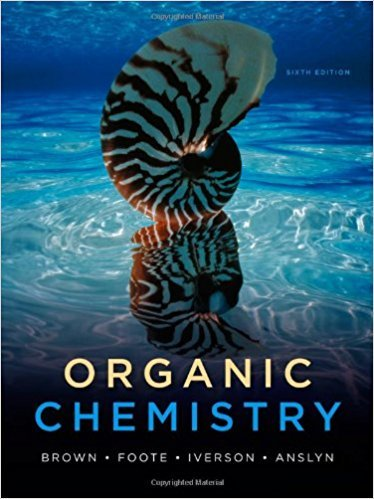×
×

# Solutions for Chapter 18: Organic Chemistry 6th Edition## Full solutions for Organic Chemistry | 6th Edition

ISBN: 9780840054982Solutions for Chapter 18

Solutions for Chapter 18
4 5 0 424 Reviews
22
4
##### ISBN: 9780840054982

Chapter 18 includes 64 full step-by-step solutions. Since 64 problems in chapter 18 have been answered, more than 42609 students have viewed full step-by-step solutions from this chapter. This expansive textbook survival guide covers the following chapters and their solutions. Organic Chemistry was written by and is associated to the ISBN: 9780840054982. This textbook survival guide was created for the textbook: Organic Chemistry, edition: 6.

Key Chemistry Terms and definitions covered in this textbook
• alkyl group

A substituent lacking p bonds and comprised of only carbon and hydrogen atoms.

• antiferromagnetism

A form of magnetism in which unpaired electron spins on adjacent sites point in opposite directions and cancel each other’s effects. (Section 23.1)

• atactic

A polymer in which the repeating units contain chirality centers which are not arranged in a pattern (they have random configurations).

The number of 12C atoms in exactly 12 g of 12C; it equals 6.022 * 1023 mol-1. (Section 3.4)

• base ionization constant (Kb).

The equilibrium constant for the base ionization. (15.6)

• Chiral center

A tetrahedral atom, most commonly carbon, that is bonded to four different groups; also called a chirality center

• cohesion.

The intermolecular attraction between like molecules. (11.3)

• dispersion forces

Intermolecular forces resulting from attractions between induced dipoles. Also called London dispersion forces. (Section 11.2)

• equilibrium-constant expression

The expression that describes the relationship among the concentrations (or partial pressures) of the substances present in a system at equilibrium. The numerator is obtained by multiplying the concentrations of the substances on the product side of the equation, each raised to a power equal to its coefficient in the chemical equation. The denominator similarly contains the concentrations of the substances on the reactant side of the equation. (Section 15.2)

• exergonic

Any process with a negative DG.

• methylene group

A CH2 group.

• nuclear model

Model of the atom with a nucleus containing protons and neutrons and with electrons in the space outside the nucleus. (Section 2.2)

• peptide bond

A bond formed between two amino acids. (Section 24.7)

• polyatomic ion

An electrically charged group of two or more atoms. (Section 2.7)

• Polyester

A polymer in which each monomer unit is joined to the next by an ester bond, as, for example, poly(ethylene terephthalate).

• Primary structure of nucleic acids

The sequence of bases along the pentose-phosphodiester backbone of a DNA or RNA molecule read from the 5’ end to the 3’ end

A radioisotope that can be used to trace the path of an element in a chemical system. (Section 21.5)

• Reaction mechanism

A step-by-step description of how a chemical reaction occurs.

• substituents

In nomenclature, the groups connected to the parent chain.